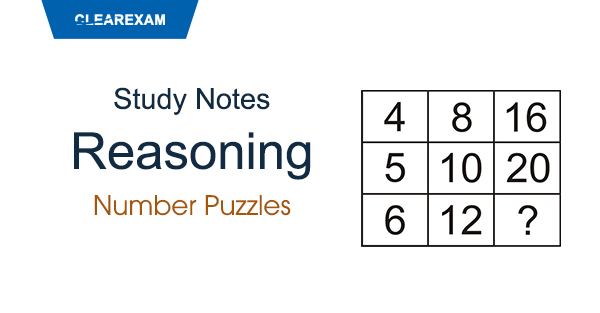## Number Puzzles

In this type of questions, a figure or a matrix is given in which some numbers are filled
according to a rule. A place is left blank. You have to find out a character
(a number or a letter) from the given possible answers which may be filled in the blank space.
Some examples are given below.
Example 1:
Which number will replace the question mark?Solution :
From fig. a: 6 + 4 + 8 = 18
18 + 2 = 20
From fig. b: 7 + 9 + 8 = 24
24 + 2 = 26
From fig. c: 6 + 5 + 12 = 23
23 + 2 = 25
Hence the number 25 will replace the question mark.
Example 2:
Which number will replace the question mark ?Solution :
From fig. a: (3)2 + (2)2 = 13
From fig. b: (4)2 + (8)2 = 80
From fig. c: ? = (1)2 + (5)2
? = 1 + 25
? = 26
Hence the number 26 will replace the question mark.
Example 3:
Which number will replace the question mark ?Solution :
From fig. a: 7 x 6 + 3 = 45
From fig. b: 5 x 4 + 6 = 26
From fig. c: 7 x 3 + 8 = 29
Hence the number 29 will replace the question mark.
Example 4:
Which number will replace the question mark ?Solution :
From fig. a: 92 + 82 + 72 + 62 = 81 + 64 + 49 + 36 = 230
From fig. b: 62 + 72 + 32 + 42 = 36 + 49 + 9 + 16 = 110
From fig. c: 92 + 62 + 52 + 42 = 81 + 36 + 25 + 16 = 158
Hence the number 158 will replace the question mark.
Example 5:
Which number will replace the question mark ?Solution :
(4 + 3)2 = (7)2 = 49
(8 + 5)2 = (13)2 = 169
(11 + 12)2 = (23)2 = 529
(10 + 9)2 = (19)2 = 361
Hence the number 361 will replace the question mark.
Example 6:
Which number will replace the question mark ?Solution :
From column I: (9 x 5) % 5 = 9
From column II: (17 x 4) % 4 = 17
From column III: (16 x ?) % 8 = 8
16? = 64
? = 4
Hence the number 4 will replace the question mark.
Example 7:
Which number will replace the question mark ?Solution :
From fig. a: (8 x 5) - (4 x 3) = 28
From fig. b: (12 x 7) - (8 x 9) = 12
From fig. c: (5 x 3) - (6 x ?) = 21
15 - 6? = 21
6? = -6
? = -1
Hence the number -1 will replace the question mark.
Example 8:
Which number will replace the question mark ?Solution :
In each row there are 'A', 'B' and 'C'
In second row 'A' and 'C' are already there
Hence in place of ?, there will be 'B'.
From first row : 4A x 6C = 24B
From third row : 9B x 4C = 36A
From second row : 5A x ? = 45C
? = (45C/5A)
? = 9B
Hence the number 9B will replace the question mark.
Example 9:
Which number will replace the question mark ?Solution :
(5)2 = 25
(6)2 = 36
(4)2 = 16
(7)2 = 49

Hence the number 49 will replace the question mark.Want to Know More
Please fill in the details below:

Name

ltr
item
Clear CTET - Coaching Institute for CTET, DSSSB and KVS: dsssb-reasoning-number-puzzle-theory
dsssb-reasoning-number-puzzle-theory